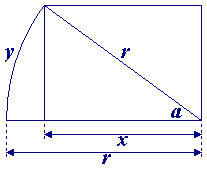## Solution to: Men on the Moon

From the first part of this puzzle, we know that the radius of the circle with a circumference of the cable's length is 1/(2×pi) meters less than the moon's radius. In the figure shown below, therefore

x = r - 1/(2×pi)

and

cos(a) = x / r = (r - 1/(2×pi)) / r

and, when taking a in radians,

y = (a / (2×pi)) × (2×pi×r) = a × r.Since r=3476000/2=1738000 meters, we can calculate that y is approximately 744 meters, which is the distance that the cable should be laid north of the moon's equator to settle the problem of the lacking 1 meter of cable.Back to the puzzle
This website uses cookies. By further use of this website, or by clicking on 'Continue', you give permission for the use of cookies. If you want more information, look at our cookie policy.